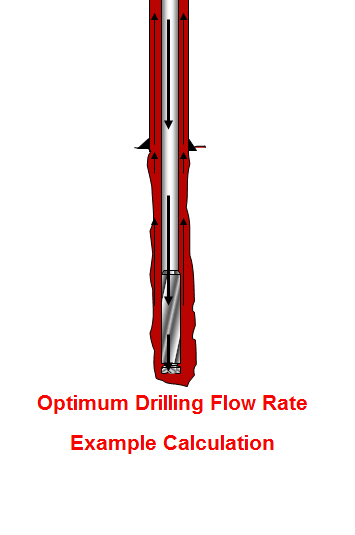## Optimum Drilling Flow Rate Example Calculation

This topic will demonstrate how to determine optimum drilling flow rate for both drilling hydraulic optimization methods (the maximum hydraulic horse power and the maximum impact force). If you don’t know the equations, please read this article first, Determine Optimum Drilling Flow Rate for Basic System – Drilling Hydraulics.The information is listed below:

Hole size = 8.5 inch

Depth In = 2,000 ft

Depth out = 6,000 ft

Mud properties at starting point

Mud weight = 9.2 ppg

PV = 13

YP = 10